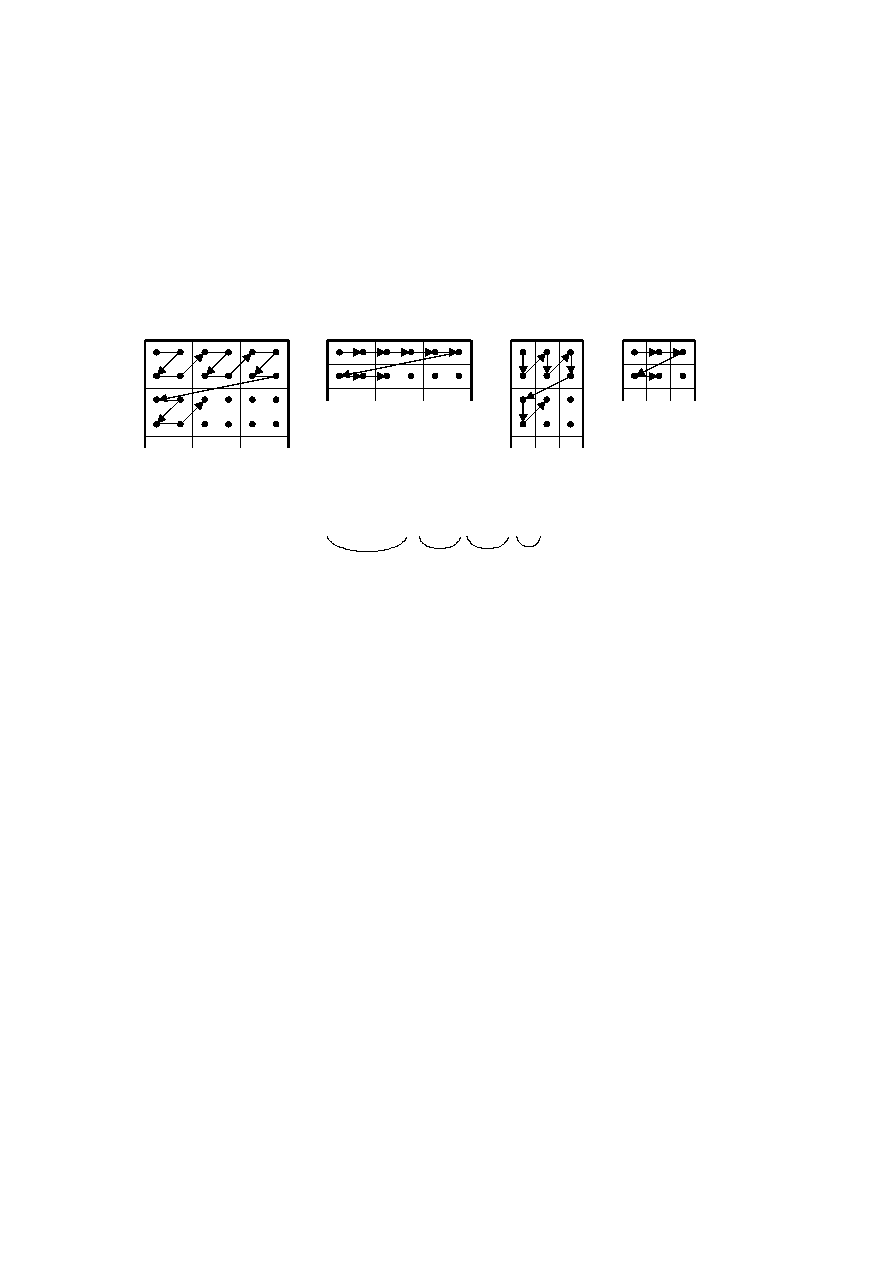ISO/IEC 10918-1 : 1993(E)
A.2.3
Interleaved order (Ns > 1)
When Ns > 1, each scan component Cs
i
is partitioned into small rectangular arrays of H
k
horizontal data units by V
k
vertical data units. The subscripts k indicate that H
k
and V
k
are from the position in the frame header component-
specification for which C
k
=
Cs
i
. Within each H
k
by V
k
array, data units are ordered from left-to-right and top-to-bottom.
The arrays in turn are ordered from left-to-right and top-to-bottom within each component.
As shown in the example of Figure A.3, Ns
=
4, and MCU
1
consists of data units taken first from the top-left-most region
of Cs
1
, followed by data units from the corresponding region of Cs
2
, then from Cs
3
and then from Cs
4
. MCU
2
follows the
same ordering for data taken from the next region to the right for the four components.
0
1
2
3
4
5
0
1
2
3
0
1
2
3
4
5
0
1
0
1
2
0
1
2
3
0
1
2
0
1
TISO0800-93/d016
Cs : H = 2, V = 2
Cs : H = 2, V = 1
Cs : H = 1, V = 2
Cs : H = 1, V = 1
1
1
1
1
2
2
2
3
3
3
4
4
4
MCU = d d d d d d d d d ,
MCU =
d d d d d d d d d ,
MCU = d d d d d d d d d ,
MCU = d d d d d d d d d ,
00
01
10
11
02
03
12
13
04
05
14
15
20
21
30
31
01
10
11
02
03
12
04
05
20
30
00
01
00
10
00
2
3
4
01
02
02
11
10
1
1
1
1
1
1
1
1
1
1
1
1
1
1
1
1
2
2
2
2
2
2
2
2
3
3
3
3
3
3
3
3
4
4
4
4
Cs data units
Cs
Cs
Cs
1
2
3
4
Figure A.3 ­ Interleaved data ordering example
FIGURE A.3 [D16] 7,5 cm = 293 %
A.2.4
Completion of partial MCU
For DCT-based processes the data unit is a block. If x
i
is not a multiple of 8, the encoding process shall extend the number
of columns to complete the right-most sample blocks. If the component is to be interleaved, the encoding process shall also
extend the number of samples by one or more additional blocks, if necessary, so that the number of blocks is an integer
multiple of H
i
. Similarly, if y
i
is not a multiple of 8, the encoding process shall extend the number of lines to complete the
bottom-most block-row. If the component is to be interleaved, the encoding process shall also extend the number of lines
by one or more additional block-rows, if necessary, so that the number of block-rows is an integer multiple of V
i
.
NOTE ­ It is recommended that any incomplete MCUs be completed by replication of the right-most column and the bottom
line of each component.
For lossless processes the data unit is a sample. If the component is to be interleaved, the encoding process shall extend
the number of samples, if necessary, so that the number is a multiple of H
i
. Similarly, the encoding process shall extend
the number of lines, if necessary, so that the number of lines is a multiple of V
i
.
Any sample added by an encoding process to complete partial MCUs shall be removed by the decoding process.
A.3
DCT compression
A.3.1
Level shift
Before a non-differential frame encoding process computes the FDCT for a block of source image samples, the samples
shall be level shifted to a signed representation by subtracting 2
P ­ 1
, where P is the precision parameter specified in B.2.2.
Thus, when P
=
8, the level shift is by 128; when P
=
12, the level shift is by 2048.
26
CCITT Rec. T.81 (1992 E)